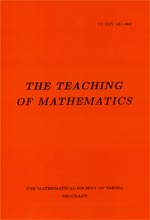﻿ The Teaching of Mathematics - Instructions for Authors ﻿
 THE TEACHING OF MATHEMATICSA reconsideration of: Number systems characterized by their operative properties'' Milosav M. Marjanović and Zoran Kadelburg AbstractThe starting point of our approach to the number systems is the selection of basic operative properties of the system $N_0$ of natural numbers with~ $0$. This set of properties has proved itself to be sufficient for extension of this system to the systems of integers $Z$, positive rational numbers $Q_+\cup\{0\}$ and rational numbers $Q$ and for the formation of basic operative properties of these extended systems. \par In all these cases of number systems, the corresponding set of numbers with basic operative properties is an example of a concrete algebraic structure. These structures can be viewed abstractly as the structure $(S, +, \cdot, <)$, where $S$ is a non-empty set, $+$'' and $\cdot$'' are two binary operations on $S$ and $<$'' is the order relation on $S$, which satisfy the postulated conditions that are formed according to the basic operative properties of these systems. When matched up with $N_0$, $Z$, $Q_+\cup\{0\}$ and $Q$, the structure $(S,+,\cdot,<)$ is called ordered semifield, ordered semifield with additive inverse, ordered semifield with multiplicative inverse and ordered field, respectively. Then, these number systems are characterized as being the smallest semifield with which they fit together. Proofs of these facts require deduction of some properties of all mentioned types of this abstract structure upon which they will be clearly relied. Hence, the main aim of this paper is this deduction and some improvements of proofs contained in the paper whose reconsideration is this note.Keywords: Number system; ordered semifield; operative property; characterization of number systems. Pages:  81$-$89 Volume  XXIII ,  Issue  2 ,  2020

﻿